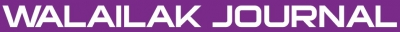A Modified Weighted Symmetric Estimator for a Gaussian First-order Autoregressive Model with Additive Outliers

Wararit PANICHKITKOSOLKUL

Abstract

Guttman and Tiao , and Chang  showed that the effect of outliers may cause serious bias in estimating autocorrelations, partial correlations, and autoregressive moving average parameters (cited in Chang et al. ). This paper presents a modified weighted symmetric estimator for a Gaussian first-order autoregressive AR(1) model with additive outliers. We apply the recursive median adjustment based on an exponentially weighted moving average (EWMA) to the weighted symmetric estimator of Park and Fuller . We consider the following estimators: the weighted symmetric estimator (), the recursive mean adjusted weighted symmetric estimator () proposed by Niwitpong , the recursive median adjusted weighted symmetric estimator () proposed by Panichkitkosolkul , and the weighted symmetric estimator using adjusted recursive median based on EWMA (). Using Monte Carlo simulations, we compare the mean square error (MSE) of estimators. Simulation results have shown that the proposed estimator, , provides a MSE lower than those of , and  for almost all situations.

Keywords

Parameter estimation, autoregressive model, recursive median, exponentially weighted moving average, additive outliers

PDF

References

I Guttman and GC Tiao. Effect of correlation on the estimation of a mean in the presence of spurious observations. Can. J. Stat. 1978; 6, 229-47.

I Chang. 1982, Outliers in Time Series, Ph. D. Dissertation. University of Wisconsin-Madison, USA.

I Chang, GC Tiao and C Chen. Estimation of time series parameters in the presence of outliers. Technometrics 1988; 30, 193-204.

HJ Park and WA Fuller. Alternative estimators and unit root tests for the autoregressive process. J. Time Ser. Anal. 1995; 16, 415-29.

S Niwitpong. 2007, Predictive Inference for Time Series, Ph. D. Dissertation. La Trobe University, Australia.

W Panichkitkosolkul. New estimator for an unknown mean Gaussian AR(1) process with additive outliers. Chiang Mai J. Sci. 2010; 37, 14-20.

B Abraham and J Ledolter. Statistical Methods for Forecasting. John Wiley & Sons, New York, 1983.

H Louni. Outlier detection in ARMA models. J. Time Ser. Anal. 2005; 29, 1057-65.

AJ Fox. Outliers in time series. J. Roy. Stat. Soc. B. 1972; 34, 350-63.

A Zaharim, R Rajali, RM Atok, K Ibrahim and AM Razali. A study on the nature of an additive outlier in ARMA(1,1) models. Eur. J. Sci. Res. 2009; 32, 362-8.

I Chang, and GC Tiao. Estimation of time series parameters in the presence of outliers. Technical Report 8, Statistics Research Center, University of Chicago, 1983.

C Chatfield. Time-Series Forecasting. Chapman & Hall, New York, 2001.

P Shaman and RA Stine. The bias of autoregressive coefficient estimators. J. Am. Stat. Assoc. 1988; 83, 842-8.

BS So and DW Shin. Recursive mean adjustment in time series inferences. Stat. Probabil. Lett. 1999; 43, 65-73.

GEP Box, GM Jenkins and GC Reinsel. Time Series Analysis: Forecasting and Control. Prentice-Hall, New Jersey, 1994.

PM Berthouex and LC Brown. Statistics for Environmental Engineers. Lewis Publishers, Florida, 2002.

A Pankratz. Forecasting with Univariate Box-Jenkins Models: Concepts and Cases. John Wiley & Sons, New York, 1983.

C Chatfield. The Analysis of Time Series: An Introduction. Chapman & Hall, New York, 2003.

R Ihaka and R Gentleman. R: A language for data analysis and graphics. J. Comput. Graph. Stat. 1996; 5, 299-314.

The R Development Core Team. An Introduction to R. R Foundation for Statistical Computing, Vienna, 2010a.

The R Development Core Team. R: A Language and Environment for Statistical Computing. R Foundation for Statistical Computing, Vienna, 2010b.

Refbacks

• There are currently no refbacks.Online ISSN: 2228-835X

http://wjst.wu.ac.th

Last updated: 17 May 2019